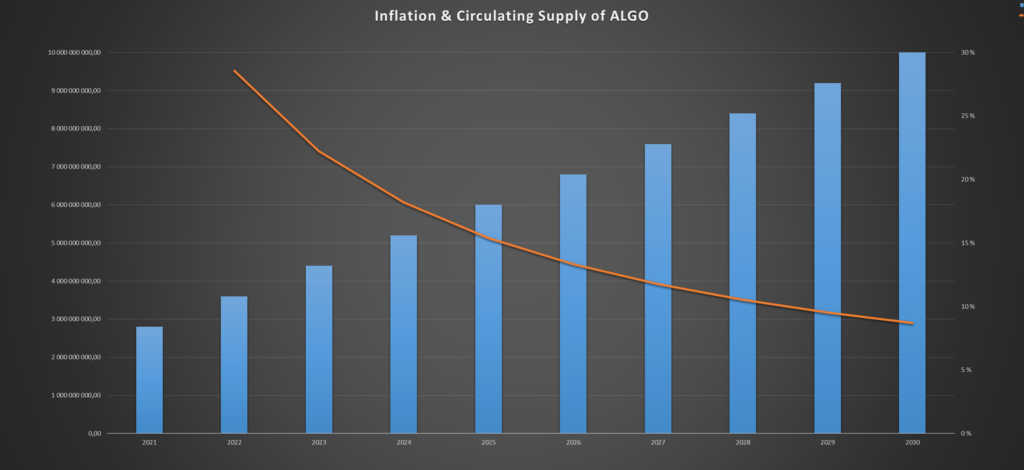# Algorand (ALGO): Inflation & Supply Mechanics

(Updated 11. September 2022)

With its amazing technology, Algorand is becoming one of the most promising projects in the blockchain space. In this article, we’ll look at the inflation rate, and the other supply mechanics of Algorands native coins, ALGO.

Here’s the short version:

The inflation rate of Algorand (ALGO) is currently 5.5% and will fall to 5% in 2023. Since the circulating supply increases linearly, the inflation rate declines exponentially. In 2030 there will be no inflation as the max supply of 10 billion ALGO is reached.

Inflation and supply mechanics are shown in graphs and diagrams below; see the table of content.

Let’s get into it, and look at the details of Algorands inflation and supply:

## The Inflation Rate of ALGO:

According to the Algorand foundation, the initial plan was to distribute all the ten billion tokens within 2024.

This would have a dramatic effect on the price. Therefore, they decided to lengthen it to 2030:

We have extended the distribution of the fixed and immutable total supply of 10bn Algo from 4 to 10 years from now, ending in 2030, rather than in 2024 as initially planned.

Algorand Foundation (2020)

Within this decade, Algorand will distribute roughly three and a half billion ALGO, increasing the supply by roughly 50%.

If we assume a constant rate of distribution, the average yearly increase in supply would be 3 500 000 000 ALGO / 9 years = 388 888 888 ALGO/year.

Even though the inflation is linear, the inflation rate would decrease annually. The circulating supply will grow each year, but the number of newly distributed coins remains constant, making the percentage increase lower every year.

### Planned Algorand (ALGO) Inflation Chart:

As you can see, the inflation rate starts out at above roughly 6%, but decreases exponentially, going below 5% in 2025.

This is a normal inflation rate for a coin like ALGO. It’s nothing huge, and will not have a huge effect on the price.

Please keep in mind that the actual inflation of ALGO isn’t linear like on the chart above. The chart above gives you the average inflation rate until the max supply is reached. It’s more likely to be logistical in nature.

If you found this last part interesting, check out this article: How the Bitcoin Halving Affects the Price of Bitcoin

The linked-to article above goes into the inflation rate of Bitcoin, and how it’s the cornerstone of Bitcoin value.

## The Supply Mechanics of Algorand (ALGO)

Supply mechanics is a big part of the equation when evaluating a coin. Let’s take a look at ALGO’s mechanic:

There are two kinds of supply; circulating supply and max supply. The circulating supply is the number of coins available on the market or in wallets. The max supply is the total coins in existence. It is the number of coins that eventually might circulate.

The circulating supply of ALGO is currently 6.9 Billion coins. However, the max supply is 10 Billion:

This means that over 3 Billion additional ALGO coins will enter circulation in the future.

“So what…?”

As you know, the price is calculated by dividing the market cap by the circulating supply.

Therefore, if the circulating supply increases, the price decreases.

From the image above, you can see that 31% of the ALGO supply is not in circulation yet.

Another way to say it is that the max supply is roughly 44% larger than the circulating supply. (Because max supply / circulating supply = 1.44)

If all these coins were to come into circulation tomorrow, how do you think this would affect the price of ALGO?

The price per token would decrease by roughly 44%.

That’s why the speed at which these coins are released, i.e the inflation rate, is highly significant and worth paying attention to.

## Summary:

The inflation rate is currently 5.5% and will decline exponentially until it stops in 2030. The circulating supply is roughly 69% of the max supply, which means that the total inflation of ALGO this decade will be 44%.